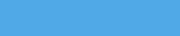# Bluish Coder

Programming Languages, Martials Arts and Computers. The Weblog of Chris Double.

2006-07-19

## Scala, futures and lazy evaluation

On the Scala wiki there is an implementation of futures, promises and lazy evaluation modelled after the same features in the Alice programming language. Implemented as a library, without changes to the Scala implementation, it seems quite seamless.

After importing the library you can create futures, etc quite easily. The examples below use this definition of the fibonacci function:

``````object FibTest {
def fib(n: int): int = n match {
case 0 => 0
case 1 => 1
case _ => fib(n-1)+fib(n-2)
}
}
``````

Lazy evaluation is done using the 'lazy' function on the Future object in scalax.futures:

``````/* Import the future names */
import Future._

/* Lazily compute the 42nd fibonacci number. The computation will
not start running until the value of 'a' is actually requested. */
var a = lazy({ FibTest.fib(42) })

/* This does not attempt to get the value of 'a' so does not start
the computation */
Console.println(a)
=> Lazy(?)

/* This will start the computation, and add 1 to the value. There is
a delay as it computes the 42nd fibonacci number. */
a+1
=> 267914297

/* No delay to compute the following as it has already been calculated */
a+2
=> 267914298
``````

Futures are different in that they immediately start computing the value in a background thread. When the value is retrieved it will block, waiting for the computation to complete, and then return the value, or return it immediately if it is available.

``````/* Compute the 42nd fibonacci number in a background thread. */
var a = spawn({ FibTest.fib(42) })

/* This will block, waiting for the computation to complete, and add 1 to the value. If it
has already completed there is no delay as it is returned immediately. */
a+1
=> 267914297
``````

The Alice programming language gives an example of an 'enum' function that returns a lazy list of incrementing integers:

``````fun enum n = lazy n :: enum (n+1)
``````

I translated this to the following Scala code:

``````/* A LazyList type that has a head and a tail. The tail being a
lazily computed lazy list itself */

/* Given a number, return a lazy list that where succesive
elements are that number incremented */
def enum(n: int): Future[LazyList[int]] = {
lazy(LazyList(n, enum(n+1)))
}

/* Notice that 'a' is a Lazy value which has not yet been computed */
var a = enum(10)
=> Lazy(?)

/* Computes the lazy value and returns the head */
=> 10

/* Display 'a' now shows that it has been partially calculated */
a
=> Lazy(LazyList(10,Lazy(?)))

/* Go further down the list... */
=> 13

/* Again we can see how much has been computed */
a
=> Lazy(LazyList(10,Lazy(LazyList(11,Lazy(LazyList(12,Lazy(LazyList(13,Lazy(?)))))))))
``````

Promises are variables with no initial value. When a thread attempts to retrieve the value they block. Some other thread can set the value of it and the original thread will then resume with that value.

``````/* Create two promises to hold integer types */
var a = promise[int]
=> Promise(?)

var b = promise[int]
=> Promise(?)

/* A future computation that uses them */
var c = spawn({ Console.println("Result is: " + (a + b)) } )
=> Spawn(?)

/* The future computation completes when 'a' and 'b' get their values */
a.set(1)
b.set(2)

/* Future completes now that it has the values it needs */
Result is: 3
``````

What is nice about the library, and that Scala allows it, is that the future values are be used exactly as if they were normal values. There is no need to call a 'get' or 'compute' to get at the value stored by the future.

A post on the Scala mailing list demonstrates how Cells style computation (automatic updating of variables when others change) can be done with the library.

### Tags

This site is accessable over tor as hidden service mh7mkfvezts5j6yu.onion, or Freenet using key:
USK@1ORdIvjL2H1bZblJcP8hu2LjjKtVB-rVzp8mLty~5N4,8hL85otZBbq0geDsSKkBK4sKESL2SrNVecFZz9NxGVQ,AQACAAE/bluishcoder/-61/

Tags

Archives